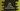# How to check if a string contains a number in Dart## Dart program to check if a string contains number:

In this post, we will learn how to check if a string contains number or not. We can use contains method to check if a string contains at least a number.

This post will show you how to use contains() method to check for any number in a string.

### contains() method:

contains method is defined as below:

``````bool contains (
Pattern other,
[int startIndex = 0]
)``````

Here, the first one is a Pattern and an optional start index. The start index is the starting index from where the search should start. In our case, we will not provide this.

For the Pattern, we can provide a regular expression, Regex that matches a string if any digit is found in it.

### Dart program:

Below dart program shows how to use contains to check if a string contains a number:

``````void main() {
var stringArr = ["Hello world", "Hello1 World", "H23llo", "H00Elo", "World"];

for (var i = 0; i < stringArr.length; i++) {
bool found = stringArr[i].contains(new RegExp(r'[0-9]'));
print(stringArr[i] + " -> " + found.toString());
}
}``````

It will print:

``````Hello world -> false
Hello1 World -> true
H23llo -> true
H00Elo -> true
World -> false``````